# Maximum Flow Problem

Use the solver in Excel to find the maximum flow from node S to node T in a directed network. Points in a network are called nodes (S, A, B, C, D, E and T). Lines in a network are called arcs (SA, SB, SC, AC, etc).

### Formulate the Model

The model we are going to solve looks as follows in Excel.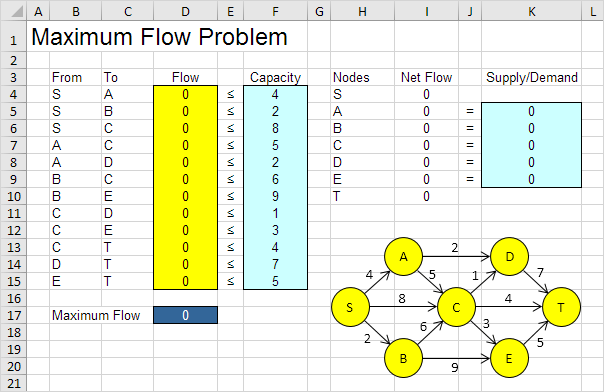1. To formulate this maximum flow problem, answer the following three questions.

a. What are the decisions to be made? For this problem, we need Excel to find the flow on each arc. For example, if the flow on SB is 2, cell D5 equals 2.

b. What are the constraints on these decisions? The Net Flow (Flow Out - Flow In) of node A, B, C, D and E should be equal to 0. In other words, Flow Out = Flow In. Also, each arc has a fixed capacity. The flow on each arc should be less than this capacity.

c. What is the overall measure of performance for these decisions? The overall measure of performance is the maximum flow, so the objective is to maximize this quantity. The maximum flow equals the Flow Out of node S.

2. To make the model easier to understand, create the following named ranges.

Range Name Cells
From B4:B15
To C4:C15
Flow D4:D15
Capacity F4:F15
SupplyDemand K5:K9
MaximumFlow D17

3. Insert the following functions.Explanation: The SUMIF functions calculate the Net Flow of each node. For node A, the first SUMIF function sums the values in the Flow column with an "A" in the From column (Flow Out). The second SUMIF function sums the values in the Flow column with an "A" in the To column (Flow In). Maximum Flow equals the value in cell I4, which is the flow out of node S. Because node A, B, C, D and E have a Net Flow of 0, Flow Out of node S will equal Flow In of node T.

### Trial and Error

With this formulation, it becomes easy to analyze any trial solution.

1. For example, the path SADT with a flow of 2. The path SCT with a flow of 4. The path SBET with a flow of 2. These paths give a total flow of 8.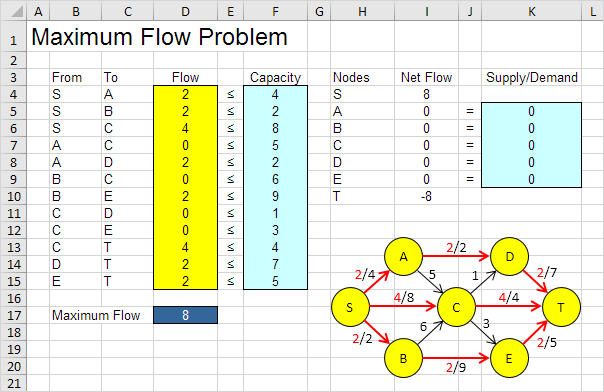It is not necessary to use trial and error. We shall describe next how the Excel Solver can be used to quickly find the optimal solution.

### Solve the Model

To find the optimal solution, execute the following steps.

1. On the Data tab, in the Analyze group, click Solver.Enter the solver parameters (read on). The result should be consistent with the picture below.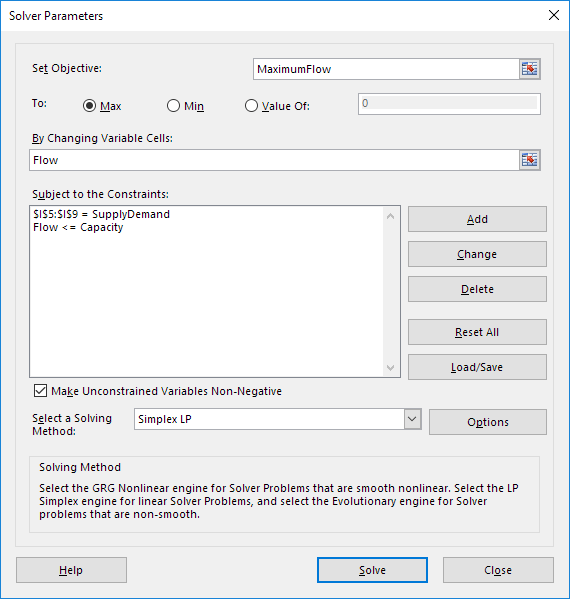You have the choice of typing the range names or clicking on the cells in the spreadsheet.

2. Enter MaximumFlow for the Objective.

3. Click Max.

4. Enter Flow for the Changing Variable Cells.

5. Click Add to enter the following constraint.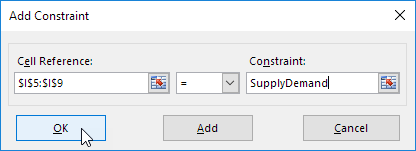6. Click Add to enter the following constraint.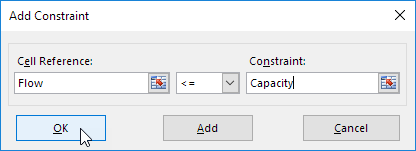7. Check 'Make Unconstrained Variables Non-Negative' and select 'Simplex LP'.

8. Finally, click Solve.

Result: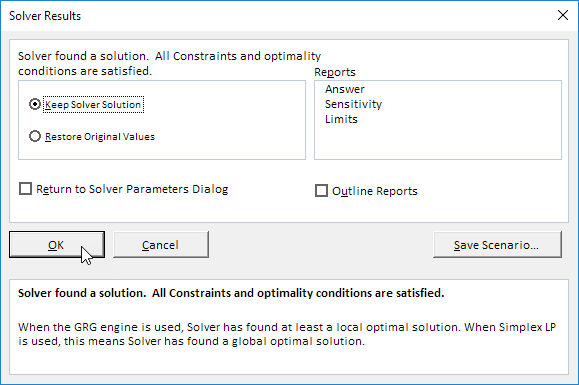The optimal solution: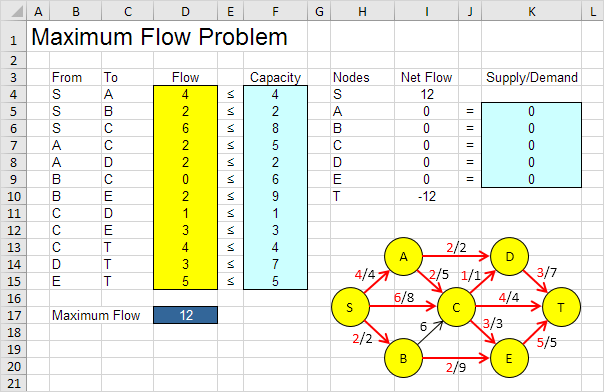Conclusion: the path SADT with a flow of 2. The path SCT with a flow of 4. The path SBET with a flow of 2. The path SCET with a flow of 2. The path SACET with a flow of 1. The path SACDT with a flow of 1. These paths give a maximum flow of 12.

Go to Next Chapter: Analysis ToolPak